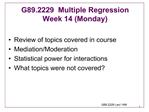# g89.2229 multiple regression week 14 monday - PowerPoint PPT PresentationDownload Presentationg89.2229 multiple regression week 14 monday

g89.2229 multiple regression week 14 mondayDownload Presentation## g89.2229 multiple regression week 14 monday

- - - - - - - - - - - - - - - - - - - - - - - - - - - E N D - - - - - - - - - - - - - - - - - - - - - - - - - - -
##### Presentation Transcript

1. G89.2229 Lect 14M Review of topics covered in course Mediation/Moderation Statistical power for interactions What topics were not covered? G89.2229 Multiple Regression Week 14 (Monday)

2. G89.2229 Lect 14M

3. G89.2229 Lect 14M Estimating Parameters Descriptive models: Fit rather than bias OLS often helpful Outliers and influential points important Must consider what is important to describe Few rigid assumptions apply Structural models Assume for unbiased estimates Properly specified model form Errorless explanatory variables Representative sample

4. G89.2229 Lect 14M Estimating Structural Models Assume for Efficient Estimates Ordinary least squares Independent observations Homoscedastic errors Few residual outliers Assume for statistical inference OLS All of assumptions listed above Residuals are normally distributed, or that sample is very large

5. G89.2229 Lect 14M Interpreting Structural Model Estimates Effect for Xj is adjusted for other variables in model The unique contribution of each variable Threats to clear interpretation Bias in estimates Model specification Selection biases not properly modeled Influential points Extrapolation rather than interpolation Fuzzy mapping of concepts to variables

6. G89.2229 Lect 14M Experimental Control vs. Statistical Control Randomized experiments

7. G89.2229 Lect 14M Tools for Making Causal Arguments Mediation Can we describe how the effect of X gets to Y? Allows tests of theories Measuring the process is difficult Usual issues in model specification Moderation Does our theory tell us when the effect of X on Y is larger or smaller? Both involve third variables

8. G89.2229 Lect 14M Baron and Kenny Mediation Steps Show that X, the independent variable, is related to Y, the dependent variable. 2. Show that X is related to M, the mediator 3. Show that M is related to Y controlling for X 4. Determine whether there is partial or complete mediation

9. G89.2229 Lect 14M Shrout and Bolger (2002) Revision For distal total effects, there may not be sufficient power to show that X->Y is different from zero (e.g. developmental examples) Using structural equation programs and "bootstrap" option, it is sometimes to show that the indirect effect is statistically significant, even if X->Y is not significant Hence, we suggest relaxing rule 1.

10. G89.2229 Lect 14M Baron and Kenny Moderation A variable W is said to moderate the relation of X to Y if the strength of the X->Y association varies with different levels of W. E.g. Free books (X) may lead to higher achievement (Y) in inner city grade schools. However, the strength of the association might vary with literacy (W) of caretaker.

11. G89.2229 Lect 14M Conceptual Refinements Kraemer et al (2002) suggest Moderating variables should be prior to causal process Distinguish from synergy, when two processes have a jointly larger effect than one alone Strongest claim for moderation is when a level of W can be found that eliminates the effect of X on Y. Rescaling of Y can sometimes eliminate apparent moderation of effects

12. G89.2229 Lect 14M Plotting and Interpreting Interaction In plots, we choose one variable for abscissa (X axis) and then represent two or three levels of moderating variable. Which variable is on abscissa can make the picture more striking.

13. G89.2229 Lect 14M Strategy for Calculating Interaction Power Use SPSS to simulate data that has a distribution that resembles predictors. Use SPSS to create outcome that follows hypothesized model Calculate R2 increase for interaction, ASSUMING NO ERROR Multiply value from step c by expected R2 for full model assuming error Use result from d with power analysis

14. G89.2229 Lect 14M Example Simulated example where Y=.4*X + .1*Z + .1*(X*Z) Plot looks striking Variance explained by interaction .029 of explained effect (X and Z uncorrelated) .024 of explained effect (Rxz=.47) If model R Square is .5, Change in R Square is .0145 or .0120 For 80% power need 330 or 410 subjects If Model R Square is .2 Change in R Square is .0058 or .0048 For 80% power need 1300 or 1550 subjects